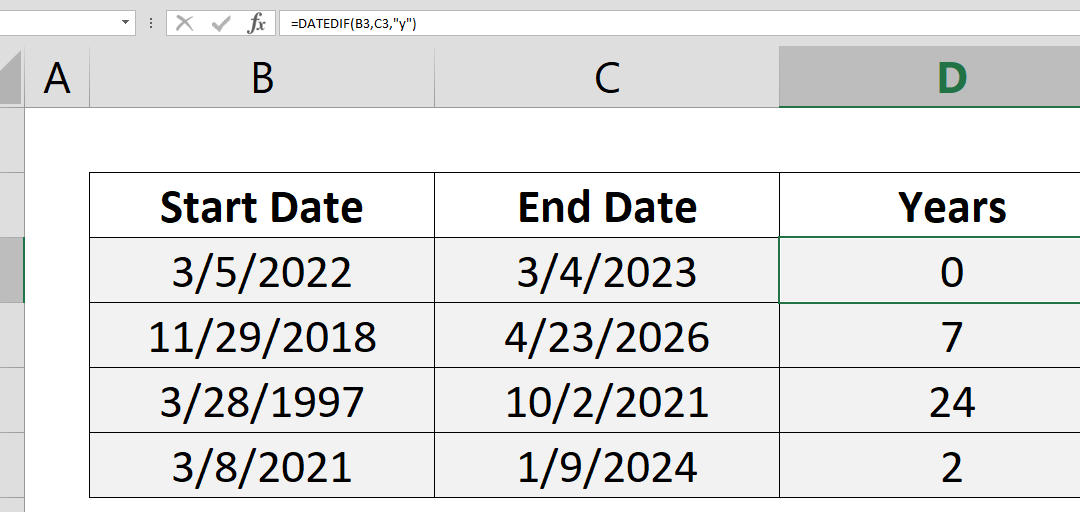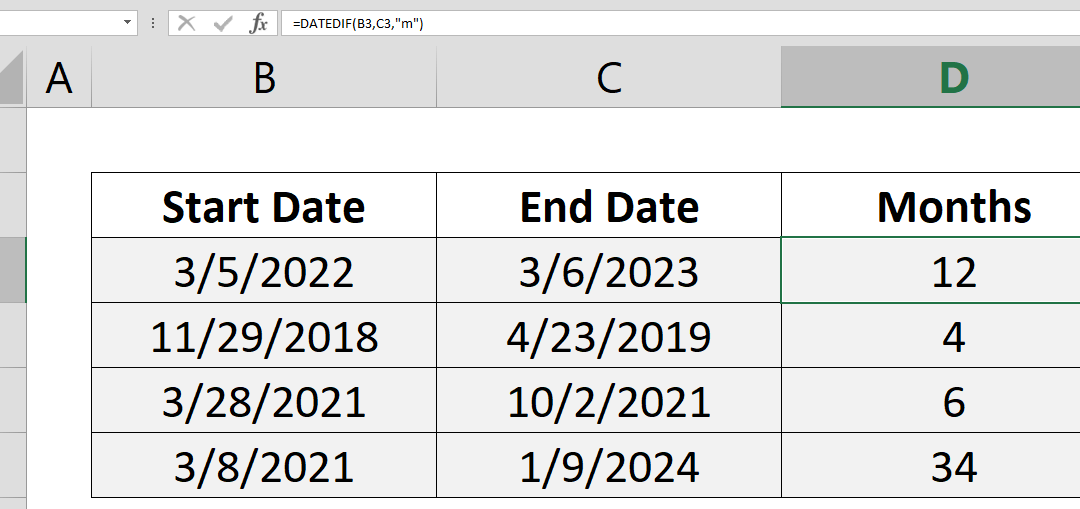Select Page## Grouping Dates in Pivot Table

Excel already has functions like the SUMIF and the SUMIFS for summing data by groups. However, they won’t work when have date-time values combined. In this article we will cover grouping dates in Pivot Table. Steps Select a cell in your data Click Pivot Table...## How to create a Quarter formula in Excel

There is no specific Quarter formula in Excel. But an Excel quarter formula can be constructed by combining ROUNDUP and MONTH functions with custom number formatting. Syntax =ROUNDUP( MONTH( date value ) /3, 0 ) Steps Start with =ROUNDUP( function Continue with MONTH(...## How to generate Excel month names

This article shows you how to generate month list by formulas and how to display Excel month names instead of actual date values. We will use DATE, YEAR and MONTH formulas as well as Number Formatting. Syntax =DATE( YEAR( previous date ), MONTH( previous date )+1, 1)...## How to find number of days in a month

This article shows you how to find number of days in a month to make your month-driven calculations dynamic. DAY and EOMONTH functions help us for this tip. Syntax =DAY(EOMONTH(Date, 0)) Steps Start with =DAY( Continue with EOMONTH( Select or type the range that...## How to count years between two dates with the DATEDIF

This article show you how to count years between two dates using the DATEDIF function. Syntax =DATEDIF(start date, end date, “y”) Steps Use =DATEDIF( Select or type range reference that includes start date B3, Select or type range reference that includes...## How to count months between two dates with the DATEDIF

This article show you how to count months between two dates using DATEDIF function. Syntax =DATEDIF(start date, end date, “m”) Steps Use =DATEDIF( Select or type range reference that includes start date B3, Select or type range reference that includes end...## How to count values if date is less than by using COUNTIF function

Using the versatile COUNTIF and COUNTIFS functions, see how you can count cells up to a certain date. How to count values if date is less than by using COUNTIF function article shows an essential tip for creating YTD (year-to-date) reports. Syntax =COUNTIFS(date...